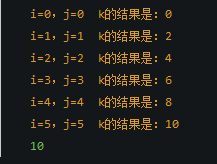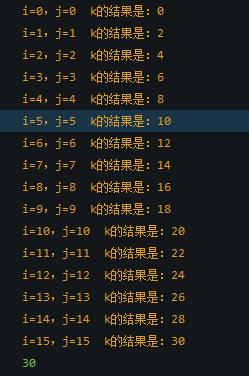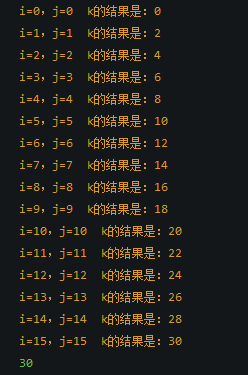# 一道js的前端面试题，主要弄清楚逗号表达式的作用

1 var i,j,k;
2 for( i = 0 , j = 0; i < 10 , j < 6; i++ , j++ ) {
3 k = i+j;
4 }
5 document.write(k); //101     for(var i = 0, j = 0; i < 10 , j < 16 ; i++ , j++){
2         k = i + j;
3         console.log('i='+i+'，j='+j+'k的结果是：'+k);
4     }
5     console.log(k);//30

控制台结果：1     for (var i = 0,j = 0; i < 16; i++, j++)
2     {
3         k = i + j;
4         console.log('i='+i+'，j='+j+'  k的结果是：'+k);
5     }
6     console.log(k);1 var t = (4,5,7); 2 console.log(t);//7

x=8*2,x*4 /*整个表达式的值为64，x的值为16*/
(x=8*2,x*4),x*2 /*整个表达式的值为128，x的值为16*/
x=(z=5,5*2) /*整个表达式为赋值表达式，它的值为10，z的值为5*/
x=z=5,5*2 /*整个表达式为逗号表达式，它的值为10，x和z的值都为5*/

###########################################################

for (i = 0; i < 10; i++, j++)
{
k = i + j;
}

console.log(k);//18

posted @ 2016-05-18 14:22  SkyTeam_LBM  阅读(...)  评论(...编辑  收藏